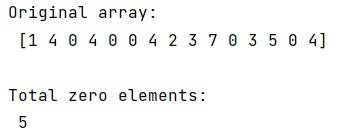# Efficiently count zero elements in numpy array

Given a NumPy array, we have to count zero elements in it.
Submitted by Pranit Sharma, on February 16, 2023

NumPy is an abbreviated form of Numerical Python. It is used for different types of scientific operations in python. Numpy is a vast library in python which is used for almost every kind of scientific or mathematical operation. It is itself an array which is a collection of various methods and functions for processing the arrays.

## Counting zero elements in numpy array

Suppose that we are given with a numpy array that contains some integer values and we need to count the zero elements. Suppose that array is not very large but the operation is to be performed thousand number of times hence, we need to find an efficient way to count the zero elements.

For this purpose, a much faster approach would be to just use np.where and pass a certain condition that arr=0 and finally take the length of the output which is equivalent of the total number of zeroes.

Let us understand with the help of an example,

## Python code to count zero elements in numpy array

```# Import numpy
import numpy as np

# Creating numpy array
arr = np.array([1,4,0,4,0,0,4,2,3,7,0,3,5,0,4])

# Display original array
print("Original array:\n",arr,"\n")

# Counting zero elements
res = np.where( arr == 0)

# Display result
print("Total zero elements:\n",len(res))
```

Output: